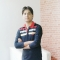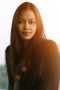## How to convert an image to a PyTorch Tensor?Updated on 06-Nov-2021 09:31:58
A PyTorch tensor is an n-dimensional array (matrix) containing elements of a single data type. A tensor is like a numpy array. The difference between numpy arrays and PyTorch tensors is that the tensors utilize the GPUs to accelerate the numeric computations. For the accelerated computations, the images are converted to the tensors.To convert an image to a PyTorch tensor, we can take the following steps −StepsImport the required libraries. The required libraries are torch, torchvision, Pillow.Read the image. The image must be either a PIL image or a numpy.ndarray (HxWxC) in the range [0, 255]. Here H, W, and ... Read More

## Delete elements with frequency atmost K in PythonUpdated on 04-May-2020 13:04:48
While manipulating data from lists we may come across scenario where we have selectively remove elements form the list based on their frequency. In this article we will explore how to remove all elements from a list whose frequency is less than equal to 2. You can also change the value 2 to any number in the programs.With countThe count methods keep the count of each element in the list. So we use it with a for loop and put a condition to only keep the elements whose count is greater than 2.Example Live DemolistA = ['Mon', 3, 'Tue', 'Mon', 9, ... Read More

## Identifying handwritten digits using Logistic Regression in PyTorch?Updated on 30-Jul-2019 22:30:25
In this we are going to use PyTorch to train a CNN to recognize handwritten digit classifier using the MNIST dataset.MNIST is a widely used dataset for hand-written classification task covering more than 70k labeled 28*28 pixel grayscale images of handwritten digits. The dataset contains almost 60k training images and 10k test images. Our job is to train a model using 60k training images and subsequently test its classification accuracy on 10k test images.InstallationFirst we need the MXNet latest version, for that just run the following on your terminal:\$pip install mxnetAnd you will something like, Collecting mxnet Downloading https://files.pythonhosted.org/packages/60/6f/071f9ef51467f9f6cd35d1ad87156a29314033bbf78ad862a338b9eaf2e6/mxnet-1.2.0-py2.py3-none-win32.whl (12.8MB) ... Read More

## Linear Regression using PyTorch?Updated on 30-Jul-2019 22:30:25
About Linear RegressionSimple Linear Regression BasicsAllows us to understand relationship between two continuous variable.Example −x = independent variableweighty = dependent variableheighty = αx + βLet's understand simple linear regression through a program −#Simple linear regression import numpy as np import matplotlib.pyplot as plt np.random.seed(1) n = 70 x = np.random.randn(n) y = x * np.random.randn(n) colors = np.random.rand(n) plt.plot(np.unique(x), np.poly1d(np.polyfit(x, y, 1))(np.unique(x))) plt.scatter(x, y, c = colors, alpha = 0.5) plt.show()OutputPurpose of Linear Regression:to Minimize the distance between the points and the line (y = αx + β)AdjustingCoefficient: αIntercept/Bias: βBuilding a Linear Regression Model with PyTorchLet's ... Read More